Hello Guest it is September 30, 2020, 07:10:43 PM

###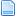Author Topic: UPDATE to Mach Specific VB routines:  (Read 6409 times)

0 Members and 1 Guest are viewing this topic.

####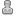poppabear••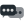2,233
• Briceville, TN, USA##### UPDATE to Mach Specific VB routines:
« on: March 31, 2009, 04:43:22 PM »
*******Last Edit 01April09, Art was Kind enough to fill in the Unknown stuff, so now the document is complete!!!**********(no not an april fools joke).

UPDATE to Mach Specific VB routines:

The currently “Undocumented”, (not on the Wiki), VB routines.
Brain sent the VB header file and I tested the ones I haven’t seen before.
There is also some “Odds and Ends” VB thrown in from various post that are useful as well.

The “Undocumented” VB:

1). System VB:

HelpAbout() //returns Mach VB script version

Random() //generates fractional random number

NotifyPlugins(int#) //a number you want to send from VB to your Plug-in, to do something in your plugin.

MachMSG( "Message",  "Title", type) //type 1-6
'int 0=OK button
'int 1= OK, CANCEL button
'int 2= Abort, Retry, Ignore buttons
'int 3= Yes, No, Cancel buttons
'int 4= Yes, No
'int 5= Rety, Cancel
'int 6= Cancel, Try Again, Continue

VerifyAxis(Silent) // is Move to zero to rehome the axis and then back to start position, no report of error or lost steps that are detected. The 1 or 0 is report or not, VerifyAxis(0) they all move to home position and then come  off of home not error reporting, and (1) will do the same plus report lost step errors.

SingleVerify(axis) //axis 0-5, Will do a VarifyAxis above except will do a Single axis and NOT report lost step errors.

SingleVerifyReport(axis) // axis 0-5, Will do a VarifyAxis above except will do a Single axis and WILL report lost step errors.

QueueDepth()  // current amount in the motion queue ( motion commands holding to be done).

IsWiz() //detects if a Wizard is running

StartVideo(); //Starts the Video Window Up.

******VBTest(); //Depreciated NO LONGER USED!!!*******

SetProbeActive(bool) // turns the probe ON or off, 1 for on, 0 for off

SetProbeState(bool) // sets the low-active on or off, 1 for on, 0 for off

CoupleSlave(bool)  //sets the slave axis to home exactly with the master axis 1 for on, 0 for off

2). Axis related:

StraightTraverse(double x, y, z, a, b, c) //move to a point shortest path

GetScale(axis) //axis 0-6

SetScale(axis,double Scale) //axis 0-6

SetMachZero(axis) // axis 0-6, this will set this to a Machine ZERO!!

GetABSPosition(axis) //axis 0-6, gets the machine coordinate of that axis

MinX(), MaxX(), MinY(), MaxY() // gets the max/min of a program in X/Y
JogOn(axis#, dir) //axis# = 0-5, dir: 1 = -, 0 = +

Jog Off(axis#) // axis# = 0-5, stops that axis from joging

RefCombination(int number)
1 = ref X
2 = ref Y
3 = ref X&Y same time,
4 = ref Z
5 = ref X&Z same time
6 = ref Y&Z same time
7 = ref X&Y&Z same time
8 = ref A
9 = ref X&A same time
10 = ref Y&A same time
11 = ref X&Y&A same time
12 = ref Z&A same time
13 = ref X&Z&A same time
14 = ref Y&Z&A same time
15 = ref X&Y&Z&A same time
16 = ref B
17 = ref X&B same time
18 = ref Y&B same time
19 = ref X&Y&B same time
20 = ref Z&B same time
21 = ref X&Z&B same time
22 = ref Y&Z&B same time
23 = ref X&Y&Z&B same time
24 = ref A&B same time
25 = ref X&A&B same time
26 = ref Y&A&B same time
27 = ref X&Y&A&B same time
28 = ref Z&A&B same time
29 = ref X&Z&A&B same time
30 = ref Z&Y&A&B same time
31 = ref X&Y&Z&A&B same time
32 = ref C
33 = ref X&C
34 = ref Y&C
35 = ref X&Y&C,
36 = ref Z&C
37 = ref X&Z&C
38 = ref Y&Z&C
39 = ref X&Y&Z&C
40 = ref A&C
41 = ref X&A&C,
42 = ref Y&A&C
43 = ref X&Y&A&C
44 = ref Z&A&C
45 = ref X&Z&A&C
46 = ref Y&Z&A&C,
47 = ref X&Y&Z&A&C
48 = ref B&C
49 = ref X&B&C
50 = ref Y&B&C
51 = ref X&Y&B&C,
52 = ref Z&B&C
53 = ref X&Z&B&C
54 = ref Y&Z&B&C
55 = ref X&Y&Z&B&C
56 = ref A&B&C,
57 = ref X&A&B&C
58 = ref Y&A&B&C
59 = ref X&Y&A&B&C
60 = ref Z&A&B&C
61 = ref X&Z&A&B&C,
62 = ref Y&Z&A&B&C
63 = ref X&Y&Z&A&B&C

GetCoord("Question") //this opens a dialog box that your question appears,
and you can enter coordinates for x, y, z and a. The next 4 functions
will get the values you have entered.

GetXCoor() //see GetCoord above

GetYCoor() //see GetCoord above

GetZCoor() //see GetCoord above

GetACoor() //see GetCoord above

SwapAxix( 2,5) //axis are 0-7, x, y, z, a, b, c, s

ResetAxisSwap()

GetDROString(axis#) //axis# 0-6, returns the axis dro value as a “String”

SetFormula(“math formula”, axis#) //this will set a “Formula” into the “Axis formula” box.

3). Tool related:

GetToolChangeStart(double) //Axis 0-6, in machine coordinates

ToolLengthOffset() //gets the offset of the current tool

SetCurrentTool(Tool#) //sets DRO 824

4). Feed Related:

CommandedFeed() //get the current Feedrate

SetFeedRate(Rate) //sets the current Feedrate

5). Spindle Related:

GetRPM() //get the programmed Spindle speed

SetSpinSpeed(rpm) //set Spindle Speed commanded DRO

SetPulley(pulley#)  //set the pulley number

6). File Related:

OpenDigFile() //Open a file last directory chosen

CloseDigFile() // opposite of above

OpenSubroutineFile(“path\to\subrouting.tap”)

7). Function Related:

THCOn() //turn on the THC output

THCOff() //turn off the THC output

StartTHC() //start THC

EndTHC() //end THC

GetLEDCondition(Led) //Led as number, returns a bool

GetFloodCond() // bool is flood on

GetMistCond() //bool is mist on

GetSpindleCond() //bool is spindle on

SetTriggerMacro(macro#) //int macro #

SetIJAbs() //set to IJ Absolute coord. sys

SetIJInc() //set to IJ Incremental coord. sys

SetIJMode(mode) //mode 0-1, 0=IJ Abs, 1=IJ inc.

GetIJMode() //returns the IJ mode, see set IJ above.

IsEstop() //estop status, 1= in estop, 0 = running

IsSafeZ()

GetMessage() //Returns the OEM error message

SetModPlugString( “message” ,cfg# , Local address#)

RetractMode() //returns the Retract mode type.

IsProbing() // returns true if in a G31 routine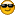. Threading Lathe Related:

tXStart()
tZStart()
tEndX()
tEndZ()
tClearX()
tSpring()
tFirstPass()
tChamfer()
tTaper()
tInFeed()
tDepthLastPass()
InFeeds(iteration) // iteration?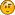ThreadDepth(iteration) // iteration?tTapers(iteration) // iteration?GetMinPass()

9). Port Related:

Writing:
Call SendFIFO("M104 S" & S$& Chr(10)& Chr(13)) Reading: D$ = GetFifoEntry()

ClearFiFo() //clears the serial FiFo buffer

SendFIFObyte(SHORT byte);

SendFIFOword(SHORT data);

10). Odds and Ends:

VB Timers //
There are 24 timers, numbered from timer 0 to timer 23.
SetTimer(x) , will reset that timer to 0.
N = GetTimer(x) , will tell you how much time has passed since it was set to
zero. Granularity is in micro seconds.

Example:
if IsActive( INPUT1 ) then
if( Timer( 1 ) > 30 ) then
Speak" 30 seconds have elapsed"
SetTimer( 1 )
endif
else
SetTimer(1)
end if

Set Trigger Macro using “Push User Button” in Brians:

First, you dont put the code in a button, you put it in a macro file. For
example file: m600.m1s
SetMessage( "This is a macro call..")

Then , you have to set that macro to be the default macro call with a call to
SetTriggerMacro( 600 ) at some point in the program, an init string could do
that if the SetTriggerMacro call were in another macro file that is called on
startup.  Then the brain will execute m600 whenever that brain termination code is
called.

To Write the Tool Table Data (and tool desc), out to a file to print:

textFilePath = "C:\mach3\ToolInfo.txt"
Set objFSO = CreateObject("Scripting.FileSystemObject")
Set objTextFile = objFSO.CreateTextFile(textFilePath)
Do Until num=225
num=num+1
N = GetToolDesc(num)
D = GetToolParam(num,1)
L = GetToolParam(num,2)
WD= Gettoolparam(num,3)
WL= Gettoolparam(num,4)
Dt= Date()
Tm= Time()

If N <>"Empty" Then

objTextFile.WriteLine("*Tool"& num &" * "& N &" *Diameter: "& D &"
*Length: "& L &" *Wear Diam"&WD &" *WearLength"&WL & Chr(13) & Chr
(10))
Else
End If
Loop

objTextFile.WriteLine("**")
objTextFile.WriteLine(" Date: " &Dt &" Time: " &Tm)

objTextFile.Close
Dim sCOMMAND As String
sCOMMAND="print c:\mach3\toolinfo.txt"
Shell("c:\winnt2\system32\cmd.exe /c" & sCOMMAND)
End

Put in #99066 = start angle for the thread in the code
so if you want to do 3 start thread then
#99066 = 0
#99066 = 120
#99066 = 240

66 is the DRO number of the start angle for a thread. When used with
#99(DRO number) the DRO is accessible in Gcode.
Sending Keys
You can easily send keys to the active window, as if the user was pressing keys on the keyboard by using the SendKeys statement.
SendKeys "This is a test string"
The code above runs notepad, and sends the keys 'This is a test string' to it. If you want to use special keys, such as  ENTER or TAB, and keys that represent actions rather than characters, use the codes shown below:
Key    Code
BACKSPACE   {BACKSPACE}, {BS}, or {BKSP}
BREAK   {BREAK}
CAPS LOCK   {CAPSLOCK}
DEL or DELETE   {DELETE} or {DEL}
DOWN ARROW   {DOWN}
END   {END}
ENTER   {ENTER}or ~
ESC   {ESC}
HELP   {HELP}
HOME   {HOME}
INS or INSERT   {INSERT} or {INS}
LEFT ARROW   {LEFT}
NUM LOCK   {NUMLOCK}
PAGE DOWN   {PGDN}
PAGE UP   {PGUP}
PRINT SCREEN   {PRTSC}
RIGHT ARROW   {RIGHT}
SCROLL LOCK   {SCROLLLOCK}
TAB   {TAB}
UP ARROW   {UP}
F1   {F1}
F2   {F2}
F3   {F3}
F4   {F4}
F5   {F5}
F6   {F6}
F7   {F7}
F8   {F8}
F9   {F9}
F10   {F10}
F11   {F11}
F12   {F12}
F13   {F13}
F14   {F14}
F15   {F15}
F16   {F16}

To specify keys combined with any combination of the SHIFT, CTRL, and ALT keys, precede the key code with one or more of the following codes:
Key    Code
SHIFT   +
CTRL   ^
ALT   %
To specify that any combination of SHIFT, CTRL, and ALT should be held down while several other keys are pressed, enclose the code for those keys in parentheses. For example, to specify to hold down SHIFT while E and C are pressed, use "+(EC)". To specify to hold down SHIFT while E is pressed, followed by C without SHIFT, use "+EC".
To specify repeating keys, use the form {key number}. You must put a space between key and number. For example, {LEFT 42} means press the LEFT ARROW key 42 times; {h 10} means press H 10 times.

Parametric Programming:

The macro code is shown in the ( ) after the macro call

Example code:

#3=3
o100
G0X#3
#3=[#3+1]
M9999 (If (GetVAR(3) > 6) Then Code" (END M9999)" Else
code"M98 P100 " )
m30
%

New Command:
(Comming out at some point after vs. 3.043.000)

CodeSingle(“G & M Code here”) //This command will be a combination of a Movement command coupled with a “While IsMoving()” built into it. Thus when you put a movement code into this, that code will complete before dropping out to the next VB code line.

‘Enjoy Scott “Poppa Bear” Shafer

« Last Edit: April 01, 2009, 10:25:02 AM by poppabear »
Commercial Mach3 & Mach 4, Design/Build/Retrofit CNC and Industrial machines.
http://www.ss-systems-llc.com/